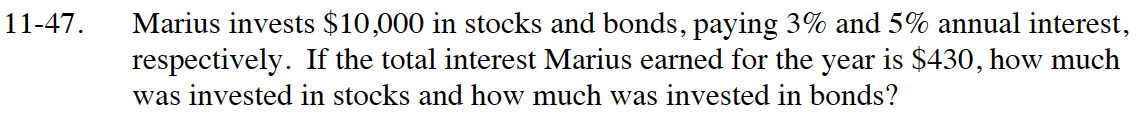### Home > CC4 > Chapter 11 > Lesson 11.2.1 > Problem11-47

11-47.

Marius invests $10,000 in stocks and bonds, paying 3% and 5% annual interest, respectively. If the total interest Marius earned for the year is$430, how much was invested in stocks and how much was invested in bonds? Homework Help ✎Let x = the amount of money invested at 3% and y = the amount of money invested at 5%. Write expressions that represent the amount of interest earned on each type of account.

Write a system of equations: one equation will be for the total amount of money invested ($10,000) the other equation will represent the interest ($430.)